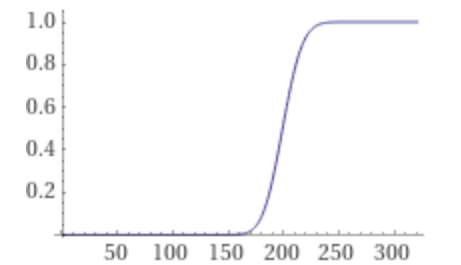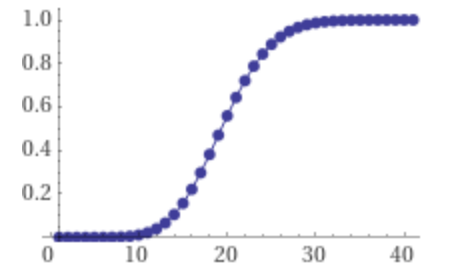# How to Estimate Max TPS from TPM

Posted on 18 Oct 2020, tagged probability theorymathtechnology

It’s good to understand the TPS (transaction per second) of a service. But sometimes we only have TPM (transaction per minute) metrics. It may because we don’t have TPS metric at all since it needs resources to compute, or it has been deleted because storing all the historical per second metrics needs a lot of storage space. So we need to estimate TPS from TPM (or even longer time period, which the method below also applies). It’s not hard to get an average TPS from TPM: just divide TPM by 60. However, because the database and the dependency services have a limit on how many concurrent requests it can handle, we also need to understand what’s the max TPS. In this article, we will explore how to do that.

We have an assumption before we go to the solution: we assume that in the time period of one minute, the requests to the service has the same probability to happen at any time. In another word, the requests are independently of the time since last request. This means the time of the requests is a uniform distribution. This is a reasonable assumption: though most services has peak requests during a day, it tends to be distributed evenly in a short period like one minute,. We need to notice that the equal of probability doesn’t mean all the requests will arrive evenly in the minute, otherwise max TPS will be the same as average TPS.

With this assumption in mind, we can use Poisson distribution to solve this problem. The probability of how many times the event occurs in the interval of time can be solved by this:

$$P(TPS=k) = \frac{\lambda^k e^{-\lambda}}{k!}$$

$$k$$ means how many times the event happens in the interval of time. $$\lambda$$ means the average of times that the event will occur in the interval.

In our case, the interval of time is 1 second. So $$\lambda$$ is the average TPS: $$TPM / 60$$. And $$P(k)$$ means the probability of the TPS during this minute.

So we have the probability of the TPS. But what we want is the max TPS. If we want to know what’s the probability of max TPS equals n, we can add all the probabilities of TPS under n:

$$P(max TPS = n) = \sum_{k=0}^{n} P(TPS=k) = \sum_{k=0}^{n} \frac{\lambda^k e^{-\lambda}}{k!}$$

Then we can draw a graph of this function and select n that makes the probability almost to 1. I recommend Wolfram Alpha to draw the graph. Though it needs paid version to show a more clear graph, the free version is enough for our use case.

Let’s give an example. Suppose we find our max TPM during peak time is 1200, then the average TPS during that minute is 200, which means $$\lambda = 200$$. Then we can draw a graph of $$P(max TPS=n)$$ with $$\lambda = 200$$:From the graph, we can see a max TPS of 260 is a safety choice. And in this minute, about 50% of the chance that the TPS will above the average TPS 200.

Sometimes the dependency has a throttling mechanism. It may has a target throttling configuration as TPS, but actually count the throttling number by sub-second metrics like transactions per 100ms. (Ideally this shouldn’t be the case but sometimes that happens and we don’t always have control over dependency services). In this case, we need to count max transactions per 100ms. Which $$\lambda = 20$$:From the graph, we can see max transactions per 100ms would be more like 36. And when we provide a target throttling TPS, we should multiply this by 10 which is 360, a lot higher than 260.

The calculation above also applies when it count throttling number independently on multiple hosts. (Again, it should have a better throttling counting mechanism). For example, if the dependency service has 10 hosts and it chooses random host to handle the request, then we should count max TPS per host. Which $$\lambda$$ is also 20 and target TPS configuration should be 360 instead of 260.

Update at Sep 22, 2022: Fix the formula from $$e^{-k}$$ to $$e^{-\lambda}$$. The graphs and results were computed with $$e{-\lambda}$$ so they are still correct.

 Prev: Understand Liveness and Fairness in TLA+ Next: Use TLA+ to Verify Cache Consistency